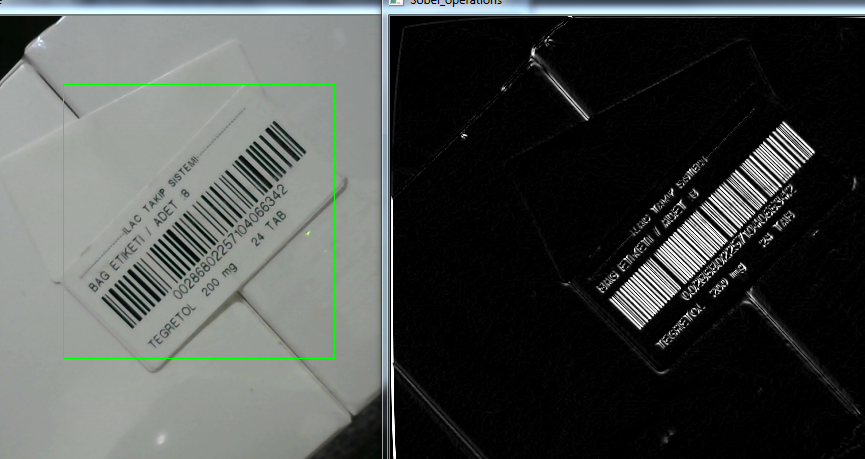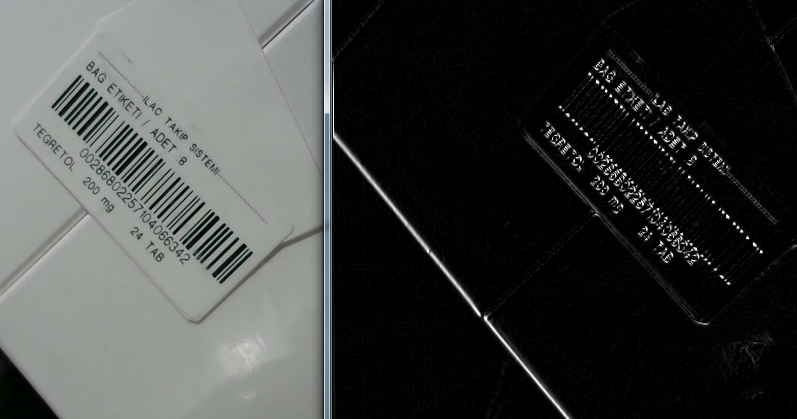Barcode recognition, Sobel derivatives and image transformation problem!

First of all, I am developing a program for barcode reading.I use Sobel derivatives to obtain Gradient representation of image in x and y direction for barcode bars.It works very well in both directions(0 to 270 degree).

But unfortunately Sobel derivative representation can not recognize another angle of the image.I don't know how to explain it, but you can understand it by looking at the two different pictures I've added below.

Correctly recognized barcode bars,Can not detect from the same angle in reverse view,Here is my code for sobel derivatives , I have added y direction gradient view to x direction using addweighted command.

vector<Sobel_variables> sobel_variables(1);
sobel_variables.alpha = 1;

sobel_variables.beta = 0.9;

Sobel(gray_image, sobel_variables.Gradx, sobel_variables.ddepth, 1, 0, 3);
Sobel(gray_image, sobel_variables.Grady, sobel_variables.ddepth, 0, 1, 3);

//sobel_variables.Out_Image.

imshow("Sobel_operations", sobel_variables.Out_Image);
return sobel_variables.Out_Image;

It is really strange.Any help will be apreciated to solve this problem.

edit retag close merge delete

if you convert the (subtracted)sobel output to CV_8U, all negative values will get saturated to 0

Thank you berak,I really apreciate your effort for my questions.But I think "convertscaleabs" function did almost the same thing that you mentioned.Unfortunately it is not effecting the result of the image.There is still the same problem.Also I changed the x and y order of the sobel function,it is affecting the result but when I solved to x order, y order gets fail and vise versea.

1

i mean, you should NOT convert it. remove the convertScaleAbs calls.

if you REALLY need a CV_8U image in the end, use normalize() with NORM_MINMAX to do that.

maybe using sobel filters is a bad idea here, because it will make "outlines" of anything. imho, you'd want "ridge detection", not "edge detection" here

I removed this convertScaleAbs as you recommended.Also I solved reverse view problem for sobel derivative with using "add" function after substraction.Should I add it with pictures as an answer? or leave it as it is.

oh, if you'd write your answer, that would be great !

Unfortunately the system does not allow me to write it. "New user" restriction

Sort by » oldest newest most voted

I solved it using "add" function to calculate image vertices in x and y axis.Therefore it we are able to combine each thresholded image in x and y direction.even if the area we want to distinguish on the image comes in the x direction, we can get the appropriate photo by combining it with the y direction.

Here is the code :

Mat Morphology::Sobel_operations(Mat& gray_image){//directly change vector with using only struct(future operations)

vector<Sobel_variables> sobel_variables(1);
//Sobel_variables sobel_variables;
sobel_variables.alpha = 1;

sobel_variables.beta = 0.9;

Sobel(gray_image, sobel_variables.Gradx, sobel_variables.ddepth, 1, 0, 3);
Sobel(gray_image, sobel_variables.Grady, sobel_variables.ddepth, 0, 1, 3);

//convertScaleAbs(sobel_variables.Gradient1, sobel_variables.Gradient1);//It can be used but not necessary

imshow("Sobel_operations", sobel_variables.Out_Image);

//------free memory of all unnecessarry images--------------------------
gray_image.release();